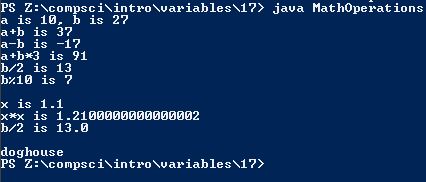# Assignment #17

## Code

```    /// Gian Adoremos
/// Period: 7
/// Program Name: Seventeenth Program
/// File Name: MathOperations.java
/// Date Finished: 9/24/2015

public class MathOperations
{
public static void main( String[] args )
{
int a, b, c, d, e, f, g;
double x, y, z;
String one, two, both;

a = 10;
b = 27;
System.out.println( "a is " + a + ", b is " + b );

c = a + b;
System.out.println( "a+b is " + c );
d = a - b;
System.out.println( "a-b is " + d );
e = a+b*3;
System.out.println( "a+b*3 is " + e );
f = b / 2;
System.out.println( "b/2 is " + f );
g = b % 10;
System.out.println( "b%10 is " + g );

x = 1.1;
System.out.println( "\nx is " + x );
y = x*x;
System.out.println( "x*x is " + y );
z = b / 2;
System.out.println( "b/2 is " + z );
System.out.println();

one = "dog";
two = "house";
both = one + two;
System.out.println( both );
}
}
```

### Picture of the output